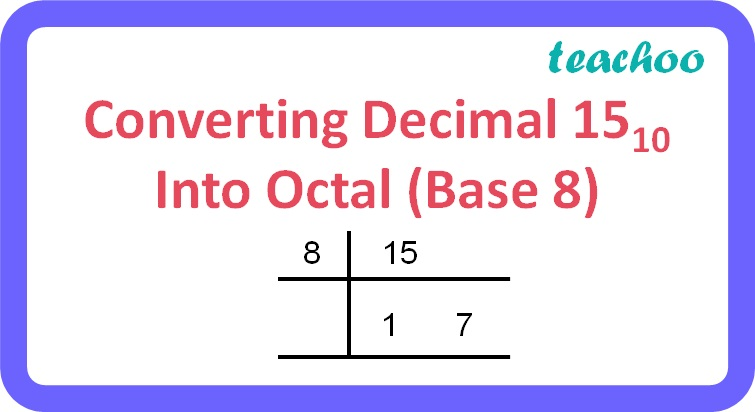MCQ questions (1 mark each)

Computer Science - Class 11
Chapter 2 Class 11 - Encoding Schemes and Number System

## (d) 21 8

15 10 is a decimal number.

To find the octal equivalent of a decimal number:

• Repeatedly divide the decimal number by 8 until the quotient becomes less than 8 and record all the remainders.
• The remainders should be written bottom to upwards to get the octal equivalent of the decimal number.Therefore, 15 10 = 17 8

So, the correct answer is (b).

Learn in your speed, with individual attention - Teachoo Maths 1-on-1 Class

### Transcript

Converting Decimal 1510 Into Octal (Base 8)